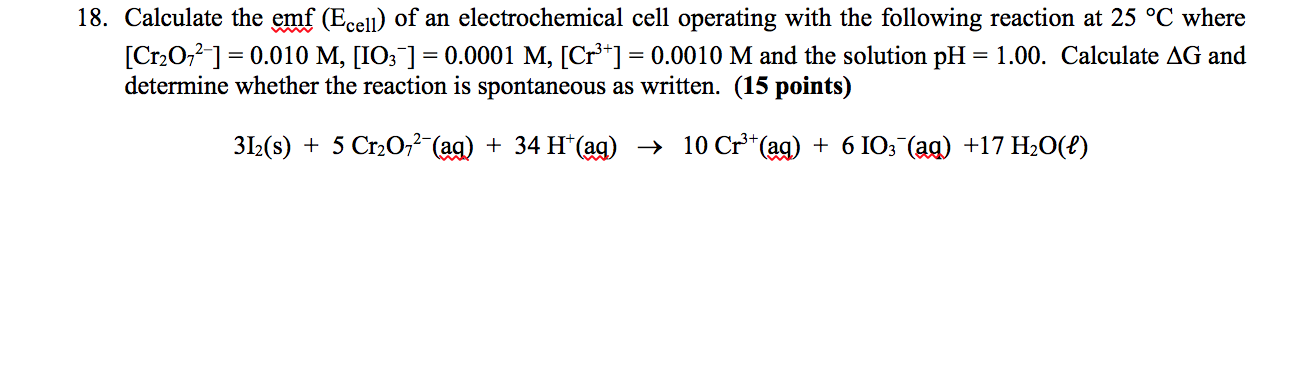# Calculate the emf (ecell) of an electrochemical cell operating with the following reaction at 25oC where [cr2o72-] =0.010 m. [IO3-] =0.0001 M, [Cr3+] =0.0010 M and the solution pH =1.00. Calculate dg and determine whether the reaction is spontaneous as written